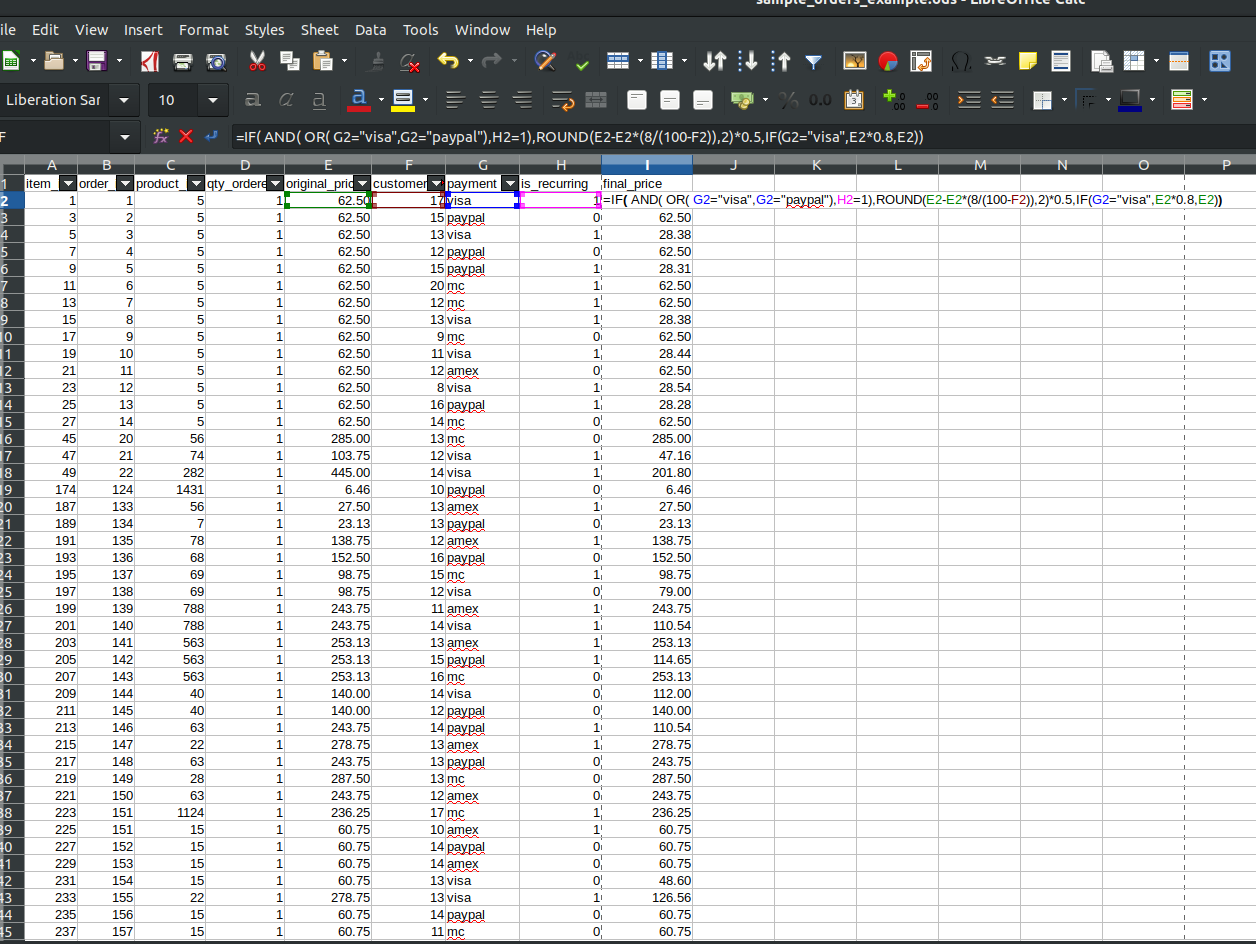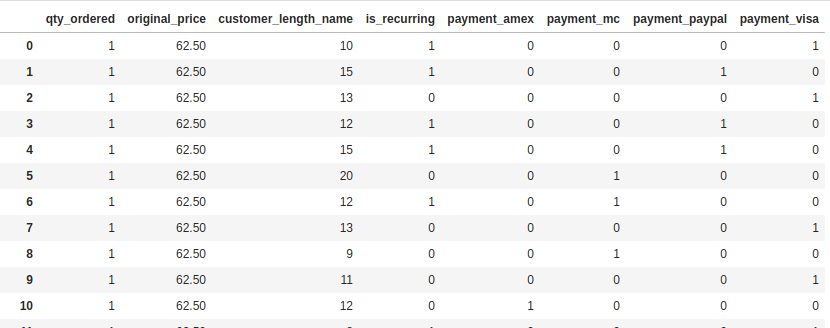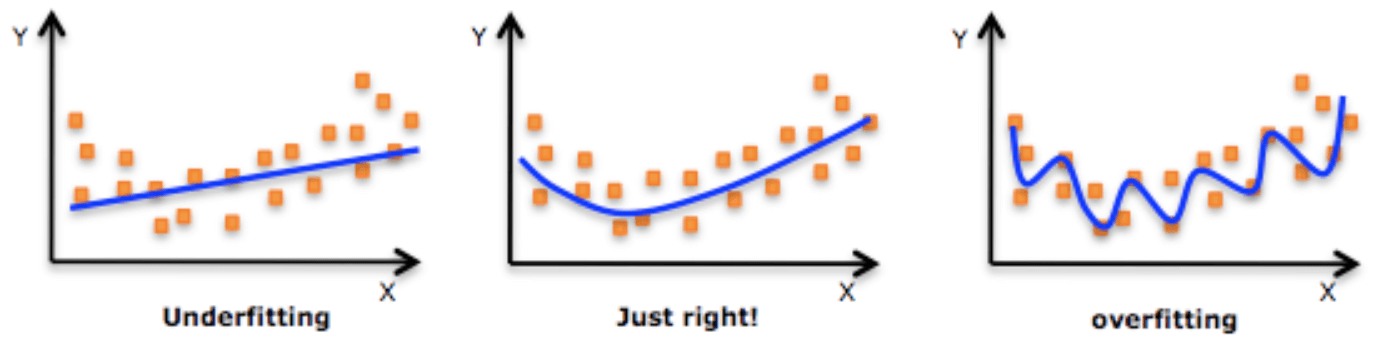Search
•Antonello Calamea

# When Magento meets Python (episode new Business Logic using ML)

A little digression before starting: I truly believe that, in few years, the approach of implementing an algorithm by code, writing it in some specific language, will be totally replaced with ML, that will figure it out during its training.

Let’s think about it: if you use ML to desume something empiric from available data (for example predicting the price of a house given its position, size, condition and so on), why not to prepare some data already having a deterministic behavior, with the goal of simply train a model and predict new values, without the need of implementing an algorithm?

Let’s see a little PoC, involving some fictional Magento raw data, to see if can work it out.

Imagine your Store Manager, wanting to implement the Perfect Promotion, a formula crafted with the most advanced tool in the universe (an excel sheet), based on particular order conditions.Following the usual way, Dev Team should first understand it, finding all the possibile outcomes and then code it, meaning days of development, testing and bug fixing.

Let’s try a ML approach with a simple (and a bit silly) example.

In this order file, the final price is calculated with a formula, based on the sum of customers first name and last name length, the payment method and if the customer is a recurring one.Don’t understand the formula? Good, you don’t need it, that’s the idea!

So, let’s train a simple regression model to have the possibility to predict new values, (hopefully) similar to the formula output.

Let’s import the data and remove unnecessary columns, then we transform the payment method in numeric values and we create two distinct sets, one for the training and one for the testing (20% of the data), with the “final_price” as the target variable (the value to predict).

```import pandas as pddf = pd.read_csv(filepath_or_buffer='sample_orders_example.csv', sep=',')df.drop(['item_id', 'order_id', 'product_id'], axis = 1, inplace=True)
from sklearn.model_selection import train_test_splitdf = pd.get_dummies(df)X = df
y = df.pop('final_price')X_train,X_test,y_train,y_test = train_test_split(X,y,test_size=0.2)X```Now let’s train the model with the test set and let’s check how it performs using some KPIs

```from sklearn.linear_model import LinearRegressionlm = LinearRegression()
model = lm.fit(X_train,y_train)y_pred_train = lm.predict(X_train)
y_pred_test = lm.predict(X_test)
def print_results(y_test, y_pred_test, model):
import numpy as np
from sklearn import metrics
results = pd.DataFrame({'Actual': y_test, 'Predicted': y_pred_test})
print(results)
print('Mean Absolute Error:', metrics.mean_absolute_error(y_test, y_pred_test))
print('Mean Squared Error:', metrics.mean_squared_error(y_test, y_pred_test))
print('Root Mean Squared Error:', np.sqrt(metrics.mean_squared_error(y_test, y_pred_test)))
results.diff = abs(results.Actual - results.Predicted)
print('Max difference €: ', max(results.diff))
print('Model score:' , model.score(X_test,y_test))

print_results(y_test, y_pred_test, model)
Actual   Predicted
380    19.89  -11.711191
232   180.13  159.477317
3273  146.88  123.158669
2005  437.50  373.705279
5692  145.87  130.505019
2581  194.00  180.675764
4715  275.00  288.327114
5119  168.43  141.841403
2334  102.89  141.466134
4174  219.38  192.582015
4921  210.00  233.558552
615   253.13  220.747508
792    10.22  -52.249891
3479   96.59  106.136767
4002   75.00  121.039295
6181  314.87  264.225071
3640   79.36   98.170555
4308  297.00  256.628011
6797   67.85  115.104304
1037  160.00  145.557379
1811  187.50  214.924272
1984   33.56   -9.829341
2337  211.65  317.382138
2321  202.50  227.648826
5882   72.67   69.591830
1100  202.50  170.099311
3870  263.25  229.161063
6761   41.23   27.728230
42     27.55    2.825145
6733   38.74   90.636408
...      ...         ...
2481  195.00  181.718931
2713   24.50    5.085685
1542  168.75  142.108454
1635   56.69   56.443899
4820  107.50   98.483982
1084  263.25  220.797198
1970  212.50  185.760688
5326  168.84  143.056744
5850  194.59  164.022063
3021   88.75   83.709668
6148  206.76  182.542196
5305  136.36  204.521611
4168   29.12  -17.508277
3514  325.00  329.704498
2230  226.88  248.137460
867   348.30  341.640514
2453  435.00  372.349412
1125  178.75  158.674934
3379  198.75  223.995417
3105  247.42  385.186108
4275  102.50   86.995270
4767   97.27  129.984805
4910  112.50  152.715481
2104  168.75  150.154966
6012  193.68  171.134513
6500  294.85  286.051647
5426  278.96  242.303499
4551  288.75  241.728520
5089  129.66  168.215840
3432  102.50   86.471361[1400 rows x 2 columns]
Mean Absolute Error: 28.99409082168446
Mean Squared Error: 1336.834584766066
Root Mean Squared Error: 36.562748594246386
Max difference €:  169.3182018272305
Model score: 0.8899216600112446```

Mmm results are not good, our Store Manager will be not happy…

This is an example of “underfitting”: our model is not performing very well with this data and, basically, it’s learning poorly.

To resolve it, we can add more info and, in this case, go “polynomial”: intuitively, imagine to have only a 2-dimensional set: this is how underfitting (and its opposite, overfitting) works.Our dataset is 9th dimensional, so it cannot be visualized, but the point is the same.

We know there is a function that will fit almost perfectly, because we created the data following a formula.

Let’s try using “PolynomialFeatures”, that will create additional features using polynomial combinations of the existing ones with degrees 2 and 3.

```from sklearn.preprocessing import PolynomialFeatures
from sklearn.pipeline import make_pipelinepipe = make_pipeline(PolynomialFeatures(3), LinearRegression())
pipe.fit(X, y)y_pred_test = pipe.predict(X_test)print_results(y_test, y_pred_test, pipe)     Actual   Predicted
380    19.89   19.913982
232   180.13  180.130583
3273  146.88  146.812533
2005  437.50  437.564871
5692  145.87  145.861954
2581  194.00  194.007231
4715  275.00  275.006263
5119  168.43  168.427097
2334  102.89  102.894418
4174  219.38  219.378466
4921  210.00  209.996858
615   253.13  253.119552
792    10.22   10.222631
3479   96.59   96.592326
4002   75.00   74.998330
6181  314.87  314.870802
3640   79.36   79.360555
4308  297.00  297.008898
6797   67.85   67.861813
1037  160.00  160.007500
1811  187.50  187.499552
1984   33.56   33.605109
2337  211.65  211.609631
2321  202.50  202.497138
5882   72.67   72.673700
1100  202.50  202.496836
3870  263.25  263.230316
6761   41.23   41.230462
42     27.55   27.531716
6733   38.74   38.775982
...      ...         ...
2481  195.00  195.007360
2713   24.50   24.643212
1542  168.75  168.747142
1635   56.69   56.678904
4820  107.50  107.488660
1084  263.25  263.263278
1970  212.50  212.495698
5326  168.84  168.853829
5850  194.59  194.596870
3021   88.75   88.812685
6148  206.76  206.759358
5305  136.36  136.421592
4168   29.12   29.123136
3514  325.00  324.986006
2230  226.88  226.886134
867   348.30  348.327729
2453  435.00  434.979682
1125  178.75  178.759465
3379  198.75  198.751748
3105  247.42  247.485879
4275  102.50  102.503684
4767   97.27   97.269478
4910  112.50  112.485685
2104  168.75  168.756693
6012  193.68  193.685397
6500  294.85  294.841766
5426  278.96  278.942916
4551  288.75  288.780942
5089  129.66  129.673586
3432  102.50  102.447167[1400 rows x 2 columns]
Mean Absolute Error: 0.022173582511703652
Mean Squared Error: 0.0011990730509815102
Root Mean Squared Error: 0.034627634209999245
Max difference €:  0.2432944290630985
Model score: 0.9999999012652929```

Bingo, almost perfect! Let’s try some predictions

```pipe.predict([[1,555,20,0,0,0,0,1]])array([443.52011166])# value from excel 444
pipe.predict([[1,555,20,1,0,0,0,1]])array([250.11617917])# value from excel 249.75```

Not bad, considering the time and the code necessary to achieve this, compared to the classic if-then approach…

See you next time!